Chapter 14 Partial Differential Equations

Physics, mechanics, engineering technology and other natural sciences often pose a large number of partial differential equation problems . Due to the needs of practice and the development of some mathematical disciplines (such as functional analysis, computing technology), the development of partial differential equation theory has been promoted, making it Form a very rich mathematics subject .

This chapter mainly introduces the theory of first-order partial differential equations, linear equations and second-order linear partial differential equations . In the second-order equations, the extremum principle, energy integration and uniqueness theorem are described . The properties and physical meanings of some solutions are clarified . , introduces the common solutions of typical elliptic, hyperbolic and parabolic equations: separation of variables method, basic solution, Green's method , Riemann's method , potential method and integral transformation method . Finally, a brief introduction to practical numerical values Solutions: Differential and Variational Methods .

§ 1 General concepts and definite solutions of partial differential equations

[ Partial differential equations and their orders ]   An equation that contains the partial derivatives of an unknown function is called a partial differential equation . If there are more than one equation, it is called a partial differential equation system . The highest order partial equation that appears in an equation or system of equations The order of the derivative is called the order of the equation or system of equations .

[ Equation Solution and Integral Surface ]   Let the function u in the region D have continuous partial derivatives of all orders that appear in the equation, if u is substituted into the equation, it can become an identity in the region D , then u is called an equation The solution in the region D , or the normal solution . It is a surface in the n + 1 -dimensional space , which is called the integral surface of the equation .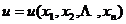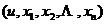[ Homogeneous linear partial differential equation and non-homogeneous linear partial differential equation ]   For the unknown function and its various order partial derivatives are linear equations are called linear partial differential equations . Such as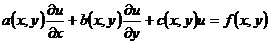is a linear equation . In a linear equation, the term that does not contain the unknown function and its partial derivatives is called the free term, such as f ( x, y ). If the free term is not zero, the equation is called inhomogeneous . If If the free term is zero, the equation is said to be homogeneous .

[ Quasilinear equation and semilinear equation ]   If an equation is linear with respect to the highest order partial derivative of the unknown function, it is called a quasilinear equation . Such as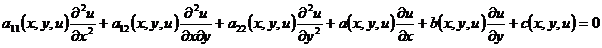is the quasi-linear equation. In the quasi-linear equation, the part composed of the highest order partial derivative is called the main part of the equation. The main part of the above equation isIf the coefficients of the main part of the equation do not contain unknown functions, it is called a semi-linear equation . For example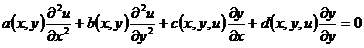is a semi-linear equation .

[ Nonlinear equation ] Equations   that are neither linear nor quasi-linear are called nonlinear equations . Such as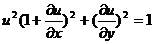It is a first-order nonlinear partial differential equation .

[ Determined solution conditions ]   Given an equation, generally only the general law of a certain motion can be described, and the specific motion state cannot be determined, so this equation is called a generalized equation . If some additional conditions (such as the known starting motion After being constrained by the outside world on the boundary), the specific motion state can be completely determined, and such a condition is called a definite solution condition . The additional condition that represents the starting situation is called the initial condition, and the condition that is constrained on the boundary is called the definite solution condition. boundary conditions .

[ Definite solution problem ] A mathematical physics problem   that gives a general definite equation (in the region D ) and the corresponding definite solution conditions is called a definite solution problem . According to different definite solution conditions, definite solution problems are divided into three categories .

The 1 ° initial value problem is a definite solution problem with only initial conditions and no boundary conditions, which is called the initial value problem or the Cauchy problem .

2 ° Boundary Value Problems A definite solution problem with only boundary value conditions and no initial conditions is called a boundary value problem .

3 ° Mixed Problems A definite solution problem with both boundary conditions and initial conditions is called a mixed problem (sometimes also called a boundary value problem) .

[ Solution of the definite solution problem ] Assuming that the   function u satisfies the generalized equation in the area D , when the point from the area D tends to give the initial value of the hyperplane or the boundary surface that tends to give the boundary conditions, in the definite solution conditions If the required limit of u and its derivative exists everywhere and satisfies the corresponding definite solution conditions, u is called the solution of the definite solution problem .

[ Solution stability ]   If a small change in the definite solution conditions only causes a small change in the solution of the definite solution problem in the entire definition domain, that is, the solution has a continuous dependence on the definite solution conditions, then the solution of the definite solution problem is said to be stable .

[ Well-posedness of the definite solution problem ]   If the solution of the definite solution problem exists and is unique and the conditions for the definite solution are stable, the formulation of the definite solution problem is said to be well-posed .